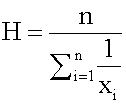Tags
#has-images #quantitative-methods-basic-concepts #statistics
Question
The harmonic mean of n numbers xi (where i = 1, 2, ..., n) is:

XH = [...]Tags
#has-images #quantitative-methods-basic-concepts #statistics
Question
The harmonic mean of n numbers xi (where i = 1, 2, ..., n) is:

XH = [...]

?

Tags
#has-images #quantitative-methods-basic-concepts #statistics
Question
The harmonic mean of n numbers xi (where i = 1, 2, ..., n) is:

XH = [...]If you want to change selection, open original toplevel document below and click on "Move attachment"

#### Parent (intermediate) annotation

Open it
Harmonic Mean The harmonic mean of n numbers x i (where i = 1, 2, ..., n) is: The special cases of n = 2 and n = 3 are given by: and so on.

#### Original toplevel document

Subject 4. Measures of Center Tendency
, therefore, is not recommended for use as the only measure of central tendency. A further disadvantage of the mode is that many distributions have more than one mode. These distributions are called "multimodal." <span>Harmonic Mean The harmonic mean of n numbers x i (where i = 1, 2, ..., n) is: The special cases of n = 2 and n = 3 are given by: and so on. For n = 2, the harmonic mean is related to arithmetic mean A and geometric mean G by: The mean, median, and mode are equal in symmetric distributions. The mean is higher than the median in positively skewed distributions and lower than the median in negatively skewed dist

#### Summary

status measured difficulty not learned 37% [default] 0

No repetitions